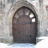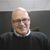Announcements
You can succeed best and quickest by helping others to succeed. Join the conversation.
cancel
Showing results for
Did you mean:Creator III

## Class function

Does anyone know if there is a way to have the buckets of a class function not repeat the right limit in the next bucket?

So lets assume the output of my class function returns the following:

0 <= x < 20

20 <= x < 40

40 <= x < 60

Is there a way that I can have the following instead?

0 <= x < 19

20 <= x < 39

40 <= x < 59

I plan to use the replace function so that I ultimately get

0 to 19

20 to 39

40 to 59

I am trying to do this in the script instead of a calculated dimension and I having to first generate a list of possible values between a min and max so I can have all the buckets appear in this field.

TIA -

1 Solution

Accepted SolutionsPartner - Champion III

What about the values 19 and 39? They are not within any of the bins?

Is it this that you want:

0 <= x <= 19  then it's the same as 0<=x<20

20 <= x <= 39

40 <= x <= 59

Then this works:

Class(Field,20)

And to make it with your logic of using to and being inclusive with lower and upper value:

SubField(Class(F,20,''),'<=  <',1)&' to '&(SubField(Class(F,20,''),'<=  <',2)-1)

3 RepliesPartner - Champion III

What about the values 19 and 39? They are not within any of the bins?

Is it this that you want:

0 <= x <= 19  then it's the same as 0<=x<20

20 <= x <= 39

40 <= x <= 59

Then this works:

Class(Field,20)

And to make it with your logic of using to and being inclusive with lower and upper value:

SubField(Class(F,20,''),'<=  <',1)&' to '&(SubField(Class(F,20,''),'<=  <',2)-1)Creator III
Author

Hi Petter,

Thanks for the reply. I didnt even think about using subfield on this so I believe that will show my desired result.

To answer your questions though, 19 and 39 were contained in the bins and it is true that the returned values of  0 <= x <20 would be the same as 0<= x <= 19, the display is what I needed to change. It is confusing to the user once you remove the <= x < signs, where the value 20 is found. Is it in the first range (now 0 to 20) or is it in the second range (20 to 40)? So that is why I needed a solution as you proposed. Thanks again.Partner - Champion III

Happy to be of helpCommunity Browser